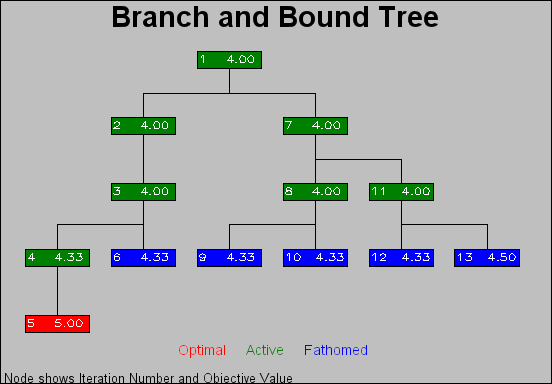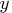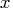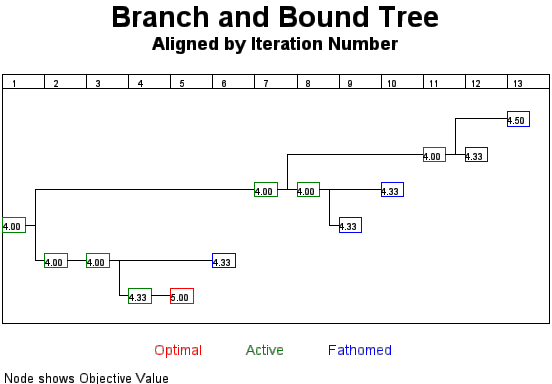Previous Page | Next Page

 The NETDRAW Procedure

## Example 7.18: Branch and Bound Trees

This example illustrates a nonstandard use of PROC NETDRAW. The TREE option in PROC NETDRAW is used to draw a branch and bound tree such as one that you obtain in the solution of an integer programming problem. Refer to Chapter 3, "The LP Procedure" (SAS/OR User's Guide: Mathematical Programming) for a detailed discussion of branch and bound trees. The data used in this example were obtained from one particular invocation of PROC LP.

The data set NET (created in the following DATA step) contains information pertaining to the branch and bound tree. Each observation of this data set represents a particular iteration of the integer program, which can be drawn as a node in the tree. The variable node names the problem. The variable object gives the objective value for that problem. The variable problem identifies the parent problem corresponding to each node; for example, since the second and the seventh observations have problem equal to '-1' and '1', respectively, it indicates that the second and the seventh problems are derived from the first iteration. Finally, the variable _pattern specifies the pattern of the nodes based on the status of the problem represented by the node.

```
data net;
input node problem cond \$10. object;
if cond="ACTIVE"          then _pattern=1;
else if cond="SUBOPTIMAL" then _pattern=2;
else                           _pattern=3;
datalines;
1       0     ACTIVE         4
2      -1     ACTIVE         4
3       2     ACTIVE         4
4      -3     ACTIVE 4.3333333
5       4 SUBOPTIMAL         5
6       3   FATHOMED 4.3333333
7       1     ACTIVE         4
8      -7     ACTIVE         4
9      -8   FATHOMED 4.3333333
10       8   FATHOMED 4.3333333
11       7     ACTIVE         4
12     -11   FATHOMED 4.3333333
13      11   FATHOMED       4.5
;
```

The next DATA step (which creates the data set LOGIC) uses this child-parent information to format the precedence relationships as expected by PROC NETDRAW. Next, the two data sets are merged together to create the Network input data set (BBTREE) for PROC NETDRAW. The ID variable in the data set BBTREE is formatted to contain both the iteration number and the objective value.

Finally, PROC NETDRAW is invoked with the TREE, ROTATE, and ROTATETEXT options to produce a branch and bound tree shown in Output 7.18.1. Note that the ROTATE and ROTATETEXT options produce a rotated graph with a top-down orientation.

```
/* set precedence relationships
using child-parent information */
data logic;
keep node succ;
set net(firstobs=2);
succ=node;
node=abs(problem);
run;

proc sort data=logic;
by node;
run;

/* combine the logic data and the node data */
/* set ID values                            */
data bbtree;
length id \$ 9;
merge logic net; by node;
if node < 10 then id=put(node,1.)||put(object,f8.2);
else              id=put(node,2.)||put(object,f7.2);
run;

goptions border;
pattern1 v=s c=green;
pattern2 v=s c=red;
pattern3 v=s c=blue;

title     j=c 'Branch and Bound Tree';
title2    ' ';
footnote1 h=1.2 j=c c=red   'Optimal  '
c=green '   Active  '
c=blue  '   Fathomed ';
footnote2 ' ';
footnote3 ' Node shows Iteration Number and Objective Value ';
proc netdraw data=bbtree graphics out=bbout;
actnet /activity=node
successor=succ
id=(id)
nodefid
nolabel
ctext=white
coutline=black
carcs=black
xbetween=15
ybetween=3
font=simplex
compress
rectilinear
tree
rotate
rotatetext
arrowhead=0
htext=2;
run;
```

Output 7.18.1: Branch and Bound TreeIn the next invocation, PROC NETDRAW uses a modified layout of the nodes to produce a diagram where the nodes are aligned according to the iteration number. The following program uses the Layout data set produced in the previous invocation of PROC NETDRAW. The samecoordinates are used; but thecoordinates are changed to equal the iteration number. Further, the ALIGN= specification produces a time axis that labels each level of the diagram with the iteration number. Each node is labeled with the objective value. The resulting diagram is shown in Output 7.18.2.

```
data netin;
set bbout;
if _seq_ = 0; drop _seq_ ;
_x_ = _from_;
id = substr(id, 3);
run;

goptions rotate=landscape;
title         'Branch and Bound Tree';
title2 h=1.5  'Aligned by Iteration Number';
footnote1 h=1.2 j=c  c=red   'Optimal     '
c=green '      Active     '
c=blue  '      Fathomed ';
footnote2 ' ';
footnote3 j=l ' Node shows Objective Value ';
pattern1 v=e c=green;
pattern2 v=e c=red;
pattern3 v=e c=blue;
proc netdraw data=netin graphics;
actnet /id=(id)
ctext=black
carcs=black
align = _from_
frame
pcompress
rectilinear
arrowhead=0
nodefid
nolabel
htext=2.5
xbetween=8;
run;
```

Output 7.18.2: Branch and Bound Tree: Aligned by Iteration NumberPrevious Page | Next Page | Top of Page